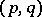# Finiteness theorems

f0403701.png ~/encyclopedia/old_files/data/F040/F.0400370 33 2 33 >>f0403706.png >>f04037035.png >>>y =(Automatically converted into $\TeX$. Above some diagnostics.)

A finiteness theorem in algebraic geometry is an assertion about the various objects in algebraic geometry (cohomology spaces, algebraic varieties, schemes, fibrations, etc.), stating that these objects depend on finitely many parameters or even form finite sets.

The first circle of finiteness theorems relates to cohomology spaces of coherent algebraic sheaves. The fundamental theorem states that these spaces are finite-dimensional over the ground field $k$ if the variety is proper (for $k = \mathbf C$ this property is equivalent to compactness) (see ). Far-reaching generalizations of this theorem have been obtained in the theory of schemes. One of them generalizes the theorem to the case of proper morphisms of schemes and asserts that the direct image of a coherent sheaf under such a mapping is coherent (see , ). Another generalization relates to the study of the cohomology of improper varieties. It turns out that if the variety $X$ under discussion is obtained by the excision of some subvariety $Y$ from a proper variety, then it is possible to estimate the dimensions in which the cohomology groups are finite-dimensional. These estimates depend on the codimension of $Y$ and on properties of its singular points (see , ). Corresponding results are known for étale cohomology.

Another circle of finiteness theorems relates to subschemes and, more generally, to coherent sheaves over a fixed proper scheme. These objects can be parametrized in a very general situation by Hilbert schemes (or by Picard schemes in the case of invertible sheaves, cf. Hilbert scheme; Picard scheme). The most general of these finiteness theorems asserts that such schemes are quasi-projective if one restricts oneself to subschemes or to sheaves with one and the same Hilbert polynomial . A special case is the fact that algebraic subvarieties of given degree in a projective space depend on finitely many parameters, as well as the theorem stating that the Néron–Severi group has a finite basis.

These theorems find application in a wide range of finiteness problems that arise in Diophantine geometry. Among them are: the question of the finiteness of the set of rational points of an algebraic variety defined over a global field (the multi-dimensional analogue of the Mordell conjecture); Shafarevich's conjecture concerning the finiteness of the number of algebraic curves defined over a given global field and with fixed degeneracies; and the question about the finite generation of the group of rational points of an algebraic group.